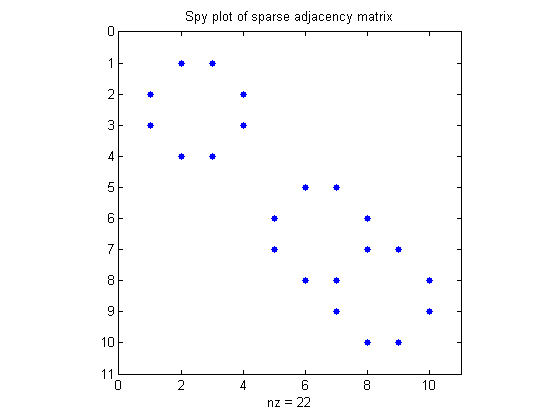# Connected component labeling – Part 3

Let's start looking at connected component labeling algorithms. In this post I want to explain how you can think of pixel neighborhood relationships in terms of a graph. We'll look at how to represent and visualize a graph in MATLAB, as well as how to compute the connected components of a graph.

Here's our sample binary image:

bw = logical([ ...
0 0 0 0 0 0 0
0 1 1 0 0 0 0
0 1 1 0 0 0 0
0 0 0 1 1 1 0
0 0 0 1 1 1 0
0 0 0 0 0 0 0 ])
bw =

0     0     0     0     0     0     0
0     1     1     0     0     0     0
0     1     1     0     0     0     0
0     0     0     1     1     1     0
0     0     0     1     1     1     0
0     0     0     0     0     0     0



Each foreground pixel becomes a node in the graph. Let's number the nodes. Theoretically, the node ordering doesn't matter very much, but for us it's convenient to use the same order as the find function:

0  0  0  0  0  0  0
0  1  3  0  0  0  0
0  2  4  0  0  0  0
0  0  0  5  7  9  0
0  0  0  6  8 10  0

Next, make a list of which nodes in the graph are connected to each other. Two nodes are connected if the corresponding pixels are neighbors. Using 4-connectivity, for example, node 1 is connected to nodes 2 and 3. Node 4 is connected to nodes 2 and 3. Let's capture these pairwise connections in a matrix:

pairs = [...
1 3; 1 2;
2 1; 2 4;
3 1; 3 4;
4 2; 4 3;
5 6; 5 7;
6 5; 6 8;
7 5; 7 8; 7 9;
8 6; 8 7; 8 10;
9 7; 9 10;
10 8; 10 9]
pairs =

1     3
1     2
2     1
2     4
3     1
3     4
4     2
4     3
5     6
5     7
6     5
6     8
7     5
7     8
7     9
8     6
8     7
8    10
9     7
9    10
10     8
10     9



From these pairs you can build up a graph representation called an adjacency matrix. An adjacency matrix has one row and column for each graph node. A nonzero element (p,q) means that there is a connection between nodes p and q. In other words, nodes p and q are adjacent. You can build up an adjacency matrix from the list of pairs by using accumarray, like this:

A = accumarray(pairs, 1)
A =

0     1     1     0     0     0     0     0     0     0
1     0     0     1     0     0     0     0     0     0
1     0     0     1     0     0     0     0     0     0
0     1     1     0     0     0     0     0     0     0
0     0     0     0     0     1     1     0     0     0
0     0     0     0     1     0     0     1     0     0
0     0     0     0     1     0     0     1     1     0
0     0     0     0     0     1     1     0     0     1
0     0     0     0     0     0     1     0     0     1
0     0     0     0     0     0     0     1     1     0



For graphs containing many nodes, you are better off constructing a sparse adjacency matrix, like this:

A = sparse(pairs(:,1), pairs(:,2), 1);
spy(A)
title('Spy plot of sparse adjacency matrix')MATLAB has a function called dmperm, which computes the Dulmage-Mendelsohn decomposition of a matrix. If the matrix is an adjacency matrix, dmperm can be used to compute the connected components of the corresponding graph. Here's how to do it.

First, you have to put 1s on the diagonal of A:

A(1:11:end) = 1;

[Note added June 29, 2007: Tim Davis pointed out this the above line is an inefficient way to get ones onto the diagonal of a sparse matrix. Please see his comments for an explanation and for better ways to do it.]

Next, call dmperm:

[p,q,r,s] = dmperm(A);

The output p contains a permuted list of nodes:

p
p =

3     4     2     1    10     9     7     8     6     5



And the output r tells you which nodes of p belong to the same connected component:

r
r =

1     5    11



Specifically, the first connected component contains these nodes:

p(r(1):r(2)-1)
ans =

3     4     2     1



The second connected component contains these nodes:

p(r(2):r(3)-1)
ans =

10     9     7     8     6     5



These two sets of nodes are the connected components of our original binary image.

Let's finish this example by using 8-connectivity instead of 4-connectivity. Our list of pairs becomes:

pairs = [...
1 2; 1 3; 1 4;
2 1; 2 3; 2 4;
3 1; 3 2; 3 4;
4 1; 4 2; 4 3; 4 5;
5 4; 5 6; 5 7; 5 8;
6 5; 6 7; 6 8;
7 5; 7 6; 7 8; 7 9; 7 10;
8 5; 8 6; 8 7; 8 9; 8 10;
9 7; 9 8; 9 10;
10 7; 10 8; 10 9];

Now repeat the steps of building the sparse adjacency matrix and calling dmperm:

A = sparse(pairs(:,1), pairs(:,2), 1);
A(1:11:end) = 1;
[p,q,r,s] = dmperm(A);
r
r =

1    11



[Note added June 29, 2007: See Tim's comment about A(1:11:end) = 1.]

Now there's only one connected component:

p(r(1):r(2)-1)
ans =

10     9     8     7     6     5     4     3     2     1



Next time we'll look at computing connected components using a kind of "flood fill" approach.

Published with MATLAB® 7.4

|